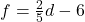## Solve for F. Show EACH step for full credit. Circle your final answer. 2d – 5f = 30 20 pts and brainliest for right answer

Question

Solve for F. Show EACH step for full credit. Circle your final answer.
2d – 5f = 30

20 pts and brainliest for right answer. pls show all work and if u can pls line it up cuz i have to write each step on paper for credit

in progress 0
6 months 2021-07-27T10:13:31+00:00 2 Answers 3 views 0

Step-by-step explanation:

2d−5f+5f=30+5f

Then,

2d=5f+30

Next,

2d/2 =5f+30/2Step-by-step explanation:

You are solving for the variable, f. Note the equal sign, what you do to one side, you do to the other. Isolate f.

Do the opposite of PEMDAS.

PEMDAS is the order of operation, and =

Parenthesis

Exponents (& Roots)

Multiplication

Division

Subtraction.

First, subtract 2d from both sides.

2d (-2d) – 5f = 30 (-2d)

-5f = -2d + 30

Next, to isolate the variable, f, divide -5 from both sides:

(-5f)/-5 = (-2d + 30)/-5

f = (-2d + 30)/-5

f = (2/5)d – 6is your answer.

Hope this helps!